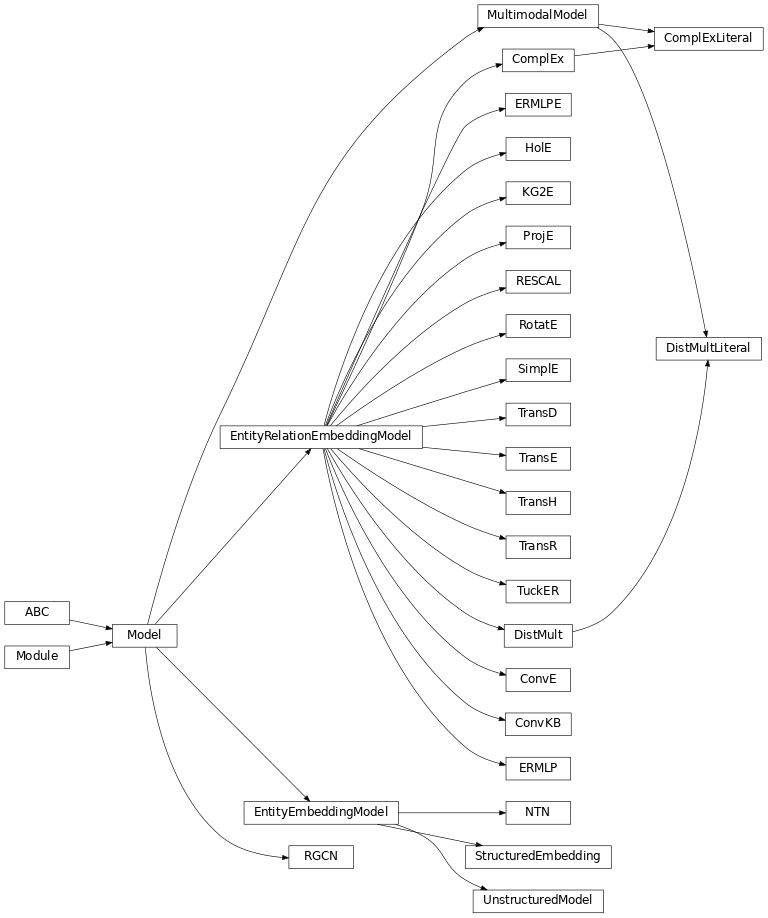# Models¶

An interaction model $$f:\mathcal{E} \times \mathcal{R} \times \mathcal{E} \rightarrow \mathbb{R}$$ computes a real-valued score representing the plausibility of a triple $$(h,r,t) \in \mathbb{K}$$ given the embeddings for the entities and relations. In general, a larger score indicates a higher plausibility. The interpretation of the score value is model-dependent, and usually it cannot be directly interpreted as a probability.

## Functions¶

 get_model_cls(query) Look up a model class by name (case/punctuation insensitive) in pykeen.models.models.

## Classes¶

 ComplEx(triples_factory[, embedding_dim, …]) An implementation of ComplEx [trouillon2016]. ComplExLiteral(triples_factory[, …]) An implementation of ComplexLiteral from [agustinus2018] based on the LCWA training approach. ConvE(triples_factory[, input_channels, …]) An implementation of ConvE from [dettmers2018]. ConvKB(triples_factory[, …]) An implementation of ConvKB from [nguyen2018]. DistMult(triples_factory[, embedding_dim, …]) An implementation of DistMult from [yang2014]. DistMultLiteral(triples_factory[, …]) An implementation of DistMultLiteral from [agustinus2018]. ERMLP(triples_factory[, embedding_dim, …]) An implementation of ERMLP from [dong2014]. ERMLPE(triples_factory[, hidden_dim, …]) An extension of ERMLP proposed by [sharifzadeh2019]. HolE(triples_factory[, embedding_dim, loss, …]) An implementation of HolE [nickel2016]. KG2E(triples_factory[, embedding_dim, loss, …]) An implementation of KG2E from [he2015]. NTN(triples_factory[, embedding_dim, …]) An implementation of NTN from [socher2013]. ProjE(triples_factory[, embedding_dim, …]) An implementation of ProjE from [shi2017]. RESCAL(triples_factory[, embedding_dim, …]) An implementation of RESCAL from [nickel2011]. RGCN(triples_factory[, embedding_dim, loss, …]) An implementation of R-GCN from [schlichtkrull2018]. RotatE(triples_factory[, embedding_dim, …]) An implementation of RotatE from [sun2019]. SimplE(triples_factory[, embedding_dim, …]) An implementation of SimplE [kazemi2018]. StructuredEmbedding(triples_factory[, …]) An implementation of the Structured Embedding (SE) published by [bordes2011]. TransD(triples_factory[, embedding_dim, …]) An implementation of TransD from [ji2015]. TransE(triples_factory[, embedding_dim, …]) TransE models relations as a translation from head to tail entities in $$\textbf{e}$$ [bordes2013]. TransH(triples_factory[, embedding_dim, …]) An implementation of TransH [wang2014]. TransR(triples_factory[, embedding_dim, …]) An implementation of TransR from [lin2015]. TuckER(triples_factory[, embedding_dim, …]) An implementation of TuckEr from [balazevic2019]. UnstructuredModel(triples_factory[, …]) An implementation of the Unstructured Model (UM) published by [bordes2014].

## Class Inheritance Diagram¶## Base Classes¶

Base module for all KGE models.

### Classes¶

 Model(triples_factory[, loss, …]) A base module for all of the KGE models. EntityEmbeddingModel(triples_factory[, …]) A base module for most KGE models that have one embedding for entities. EntityRelationEmbeddingModel(triples_factory) A base module for KGE models that have different embeddings for entities and relations. MultimodalModel(triples_factory[, loss, …]) A multimodal KGE model.

## Initialization¶

Embedding weight initialization routines.

init_phases(x)[source]

Generate random phases between 0 and $$2\pi$$.

Return type

Tensor

xavier_normal_(tensor, gain=1.0)[source]

Initialize weights of the tensor similarly to Glorot/Xavier initialization.

Proceed as if it was a linear layer with fan_in of zero and Xavier normal initialization is used. Fill the weight of input embedding with values values sampled from $$\mathcal{N}(0, a^2)$$ where

$a = \text{gain} \times \sqrt{\frac{2}{\text{embedding_dim}}}$
Parameters
Return type

Tensor

Returns

Embedding with weights by the Xavier normal initializer.

xavier_uniform_(tensor, gain=1.0)[source]

Initialize weights of the tensor similarly to Glorot/Xavier initialization.

Proceed as if it was a linear layer with fan_in of zero and Xavier uniform initialization is used, i.e. fill the weight of input embedding with values values sampled from $$\mathcal{U}(-a, a)$$ where

$a = \text{gain} \times \sqrt{\frac{6}{\text{embedding_dim}}}$
Parameters
• tensor – A tensor

• gain (float) – An optional scaling factor, defaults to 1.0.

Returns

Embedding with weights by the Xavier uniform initializer.

## Extra Modules¶

PyKEEN internal “nn” module.

class Embedding(num_embeddings, embedding_dim, initializer=None, initializer_kwargs=None, normalizer=None, normalizer_kwargs=None, constrainer=None, constrainer_kwargs=None, trainable=True)[source]

Trainable embeddings.

This class provides the same interface as torch.nn.Embedding and can be used throughout PyKEEN as a more fully featured drop-in replacement.

Instantiate an embedding with extended functionality.

Parameters
property embedding_dim

The representation dimension.

Return type

int

forward(indices=None)[source]

Get representations for indices.

Parameters

indices (Optional[LongTensor]) – shape: (m,) The indices, or None. If None, return all representations.

Return type

FloatTensor

Returns

shape: (m, d) The representations.

get_in_canonical_shape(indices=None)[source]

Get embedding in canonical shape.

Parameters

indices (Optional[LongTensor]) – The indices. If None, return all embeddings.

Return type

FloatTensor

Returns

shape: (batch_size, num_embeddings, d)

classmethod init_with_device(num_embeddings, embedding_dim, device, initializer=None, initializer_kwargs=None, normalizer=None, normalizer_kwargs=None, constrainer=None, constrainer_kwargs=None)[source]

Create an embedding object on the given device by wrapping __init__().

This method is a hotfix for not being able to pass a device during initialization of torch.nn.Embedding. Instead the weight is always initialized on CPU and has to be moved to GPU afterwards.

Return type

Embedding

Returns

The embedding.

property num_embeddings

The total number of representations (i.e. the maximum ID).

Return type

int

post_parameter_update()[source]

Apply constraints which should not be included in gradients.

reset_parameters()[source]

Reset the module’s parameters.

Return type

None

class RepresentationModule[source]

A base class for obtaining representations for entities/relations.

Initializes internal Module state, shared by both nn.Module and ScriptModule.

forward(indices=None)[source]

Get representations for indices.

Parameters

indices (Optional[LongTensor]) – shape: (m,) The indices, or None. If None, return all representations.

Return type

FloatTensor

Returns

shape: (m, d) The representations.

post_parameter_update()[source]

Apply constraints which should not be included in gradients.

reset_parameters()[source]

Reset the module’s parameters.

Return type

None